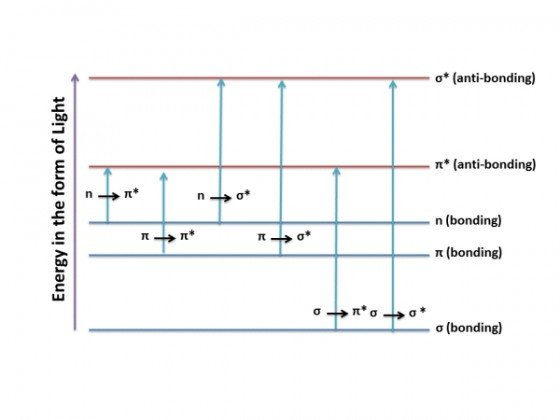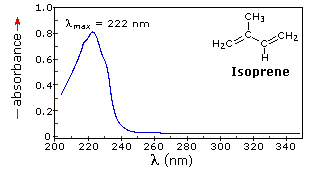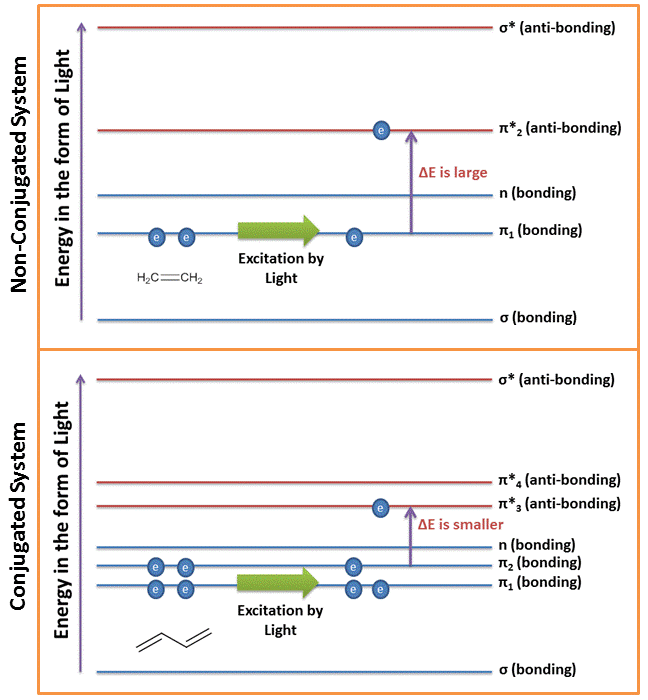# Introduction

In our discussion in “Introduction to the Electromagnetic Spectrum and Spectroscopy” we have discussed the different wavelengths for ultraviolet and visible lights which range from 10 nm to 400nm and 400nm to 780 nm respectively. The following chapter discusses to a greater extent the principles involved in the utility of ultraviolet-visible spectroscopy (UV-Vis) and the Beer-Lambert law which is useful in quantitative analysis of samples.

# Principle of Ultraviolet-Visible Absorption

As was seen in the chapter for the “Introduction to the Electromagnetic Spectrum and Spectroscopy”, the energy of the radiation can be calculated by the equation:
E = h . ν
Thus the energy of the radiation in the visible range is generally: 36 to 72 kcal/mole while that in the ultraviolet range goes as high as 143 kcal/mole. This energy irradiated on the molecules can result in changes in the electronic nature of the molecule i.e. changes between ground state and excited states of electrons within the system. As a result, UV-visible spectroscopy is also known as electronic spectroscopy.

Every time a molecule has a bond, the atoms in a bond have their atomic orbitals merged to form molecular orbitals which can be occupied by electrons of different energy levels. Ground state molecular orbitals can be excited to anti-bonding molecular orbitals.

The electrons in a molecule can be of one of three types: namely σ (single bond), π (multiple-bond), or non-bonding (n- caused by lone pairs). These electrons when imparted with energy in the form of light radiation get excited from the highest occupied molecular orbital (HOMO) to the lowest unoccupied molecular orbital (LUMO) and the resulting species is known as the excited state or anti-bonding state.

1. σ-bond electrons have the lowest energy level and are the most stable electrons. These would require a lot of energy to be displaced to higher energy levels. As a result these electrons generally absorb light in the lower wavelengths of the ultraviolet light and these transitions are rare.
2. π-bond electrons have much higher energy levels for the ground state. These electrons are therefore relatively unstable and can be excited more easily and would require lesser energy for excitation. These electrons would therefore absorb energy in the ultraviolet and visible light radiations.
3. n-electrons or non-bonding electrons are generally electrons belonging to lone pairs of atoms. These are of higher energy levels than π-electrons and can be excited by ultraviolet and visible light as well.

Most of the absorption in the ultraviolet-visible spectroscopy occurs due to π-electron transitions or n-electron transitions. Each electronic state is well defined for a particular system i.e. a double bond in 2-butene would have a particular energy level for the π-electons which when absorbs a specific (or quantized) amount of energy would get excited to the π* energy level for the electrons.

The figure below shows the different transitions between the bonding and anti-bonding electronic states.Different transitions between the bonding and anti-bonding electronic states when light energy is absorbed in UV-Visible Spectroscopy.

When a sample is exposed to light energy that matches the energy difference between a possible electronic transition within the molecule, a fraction of the light energy would be absorbed by the molecule and the electrons would be promoted to the higher energy state orbital. A spectrometer records the degree of absorption by a sample at different wavelengths and the resulting plot of absorbance (A) versus wavelength (λ) is known as a spectrum. The wavelength at which the sample absorbs the maximum amount of light is known as λmax. For example, shown below is the spectrum of isoprene. Isoprene is colorless as it does not absorb light in the visible spectrum, and has a λmax of 222nm.UV-visible spectrum of isoprene showing maximum absorption at 222 nm.

## Chromophore

Certain chemical groups or entitities are susceptible to absorb light due to the electronic configuration of the electrons in the functional group. These groups are known as chromophores. For example, the table below lists commonly found chromophores and their estimated absorbances.

 Chromophore Example Excitation λmax (nm) Solvent C = C Ethene π → π* 171 Hexanes C = O Ethanal π → π* n → π* 180 290 Hexane N = O Nitromethane π → π* n → π* 200 275 Hexane

## Effect of Conjugation

Conjugation of π-electrons affects the energy levels of the π-electrons. When two double bonds are conjugated, the electrons in them create four molecular orbitals (i.e. two bonding and two anti-bonding). See the figure below. As a result of this the highest occupied molecular orbital (HOMO) is at a higher energy state and the lowest unoccupied molecular orbital (LUMO) is of at a lower energy state. In order to excite this system, the energy that would be required to excite the electrons from the HOMO to the LUMO would therefore be reduced. As a result of this reduction in energy levels, the wavelength for absorption of conjugated molecules increases.Diagram shows how a non-conjugated system would require more energy for absorption as compared to conjugated system.

## Terminology for Absorption Shifts

 Nature of the Shift Descriptive Term To Greater Absorbance Hyperchromic To Lesser Absorbance Hypochromic To Longer Wavelength Bathchromic or Red Shift To Shorter Wavelength Hypsochromic or Blue Shift

## Why Ultraviolet-Visible Spectra are not Sharp?

Between the different electronic energy levels are the vibrational energy levels caused due to vibrational changes within the system. It must be remembered that UV-visible light can excite molecular vibrational levels as well. As a result of this phenomenon, there is not one sharp peak obtained in the UV-Visible spectra, but rather a smooth curve shaped peak for absorption as will be seen in several examples.Vibrational energy levels cause ultraviolet-visible spectra to be smooth and not sharp peaks.

## Books on Analytical Chemistry and Spectroscopy

Check out these good books for analytical chemistry and spectroscopy

# References

1. Analytical Chemistry: An Introduction (Saunders Golden Sunburst Series) 7th Ed., by Douglas A. Skoog, Donald M. West, F. James Holler. 1999.
2. Fundamentals of Analytical Chemistry 8th Ed., by Douglas A. Skoog, Donald M. West, F. James Holler, Stanley R. Crouch. 2003.
3. MSU Chemistry – UV-Visible Spectroscopy

#### Related Posts

1.2.